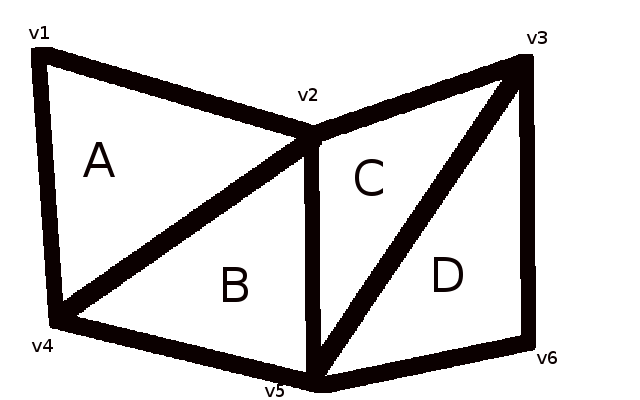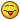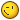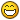# the mystery of computeNormals

## Recommended Posts

Hi,

As some of you (the most curious) asked me why I prefered to implement a dedicated normal computation for the Cylinder, I'll try to explain this with some PG.

Note : all I'll show here with a ribbon is the same with any mesh built with the same way, it is to say some laces between two pools of vertices (plane, ground, etc). And it only concerns some vertices on the edges (if any), those belonging to 3 faces only.

Let's go.

Here is a flat ribbon : http://www.babylonjs-playground.com/#G6DG0

As you can see, I used a Jahow's function to show you its normals what are computed with computeNormals() like for many meshes (all parametric shapes actually)

There's nothing mysterious here, right ?

Let's have a look to how the ribbon is built : http://www.babylonjs-playground.com/#G6DG0#1

There are 3 vertices on each horizontal edge. They are linked by groups of three to construct triangles (faces)

Thus there are 4 successive triangles stuck together from left to right. Right ?

So the vertex in the middle of the top edge belongs to the 3 first left triangles. The same for the vertex in the middle of the bottom edge.

The other vertices belong to only one or two triangles.

Please take the time to check and understand this because this is the key of the mystery !

Let's now morph a bit this ribbon by giving each vertex a z coordinate value : http://www.babylonjs-playground.com/#G6DG0#2

Have accurately a look to each normal. Nothing weird ?

Look carefully at the normals on the middle vertices. They aren't the same orientation !

Check with real lighting : http://www.babylonjs-playground.com/#G6DG0#3

You can also notice the specular isn't "right" on each plane part of the ribbon.

Why ?

Before going further, I will try to explain here how the BJS computeNormals() works. I said BJS computeNormal(), but the ThreeJS' one does exactly the same in their algorithm. The differences between the two frameworks are just the way this algo is implemented and what data structures are used : we chose the less object allocations (low level assignations) and the less passes to focus on performance rather than code elegance here.

So BJS computeNormals() is quite usable in the render loop.

The concept is : there is a normal vector per vertex.

So how can we compute it ?

As we know that a face is a triangle (we just need 3 points in space to define a plane), this means a face is defined by three vertices, let's call them p1, p2 and p3.

We can then depict the sides of this triangle by 3 vectors : p1p2, p2p3 and p1p3.

Remember your (distant for me) maths courses : if you calculate the cross product of two vectors, you get another vector orthogonal to the two initial vectors. This means orthogonal to the plane defined by these two initial vectors.

So Cross(p1p2, p2p3) will give a vector orthogonal to p1p2 and p2p3. It is to say a vector orthogonal to the triangle, so to the face.

That is just what computeNormals does.

It iterates on each face of a mesh and compute for each one a cross product and then normalize it.

Well, until now we just have normals per face, not per vertex.

So computeNormals does something more : it assigns the cross product of a face to each vertex of this face.

In our example, the (normalized) computed cross product of the triangle p1p2p3 would be the normal of p1, the normal of p2 and the normal of p3.

Nothing difficult until now.

But remember our ribbon in the PG : some vertices belong to many faces. So what are their normals ?

ComputeNormals() does another little thing : if a vertex belongs to several faces, then the sum of the normal vectors of each face is assigned to this vertex, then normalized. This allow to the light reflection to take in account consecutive faces and to usually render a smooth specular between different faces.

In brief, each face of a common vertex gives its weight in this vertex normal computation.

This works pretty well anywhere in the general case.

Let's go back to our ribbon : http://www.babylonjs-playground.com/#G6DG0#1

Let's give each triangle a name from the left to the right : A, B, C, D

And let's give each vertex a number from the left to the right : v1, v2, v3 for the upper edge and v4, v5, v6 for the lower one.Now let's have a look at the vertex v2 (in the middle top) : v2 belongs to the faces A, B and C.

Don't go further while you can't see that, just check again.

So v2 is a part a three different faces. Its normal will be the sum of the normals of the faces A, B and C. Right ?

As long as A, B and C are aligned on the same plane (the flat ribbon here), they all have the same normals : vectors orthogonal to their common plane.

Now, what append if we bend the ribbon : http://www.babylonjs-playground.com/#G6DG0#2  ?

The normal of v2 is still computed by addind the (normalized) normals of the faces A, B and C.

As A and B are on the same plane (they look like a quad) on the left of v2, they still both have the same normals.

C is a triangle, on the right of v2, it has its own different normal what is the same than the D one because C and D are on the same plane (looking like another quad).

However, the normal of D isn't taken in account in the v2 normal computation because v2 doesn't belong to D. Ok ?

Thus, the v2 normal is just the vector sum of A normal, B normal and C normal.

That's why the v2 normal is oriented to the left of v2 since A and B are on the left of v2.

That's not a bug. It's only the general way the normals are computed per vertex !

As A and B look like a quad (AB) and C and D look like another quad (CD), human eyes (I assume only human beings read this) expect the light reflection to be the same on the AB quad and on the CD quad.

If C and D weren't a plane quad, you wouldn't have notice something weird. Maybe the same if A and B weren't a plane quad either.

We just meet here a very particular case : a vertex common only to 3 faces and these faces belonging to 2 different quads each one on a different plane.

Indeed, if we add several more vertices to this ribbon, what can we see ?  http://www.babylonjs-playground.com/#G6DG0#4

We can see the great majority of the normals are like our eyes expect and the light reflection isn't that weird : http://www.babylonjs-playground.com/#G6DG0#5

This ribbon is not that big yet, only 100 vertices. Imagine when you use a 10K vertices ribbon, nothing is then noticeable anymore.

Why are all these normals "better" ?

Look back at the wireframe : http://www.babylonjs-playground.com/#G6DG0#4

Almost all the vertices belong to an even number of faces (2, 4 or 6) and these faces are then symetric to each other around the concerned vertex. In other words, the normal weights of face of a given vertex balance each other.

Only the few vertices on the edge belonging only to 3 faces still have the "weird" normals.

Capice ?

simple, isn't it.Let's now considerer how many passes and comparisons would necessary to computeNormals if it had to check if each triangular face is on the same plane than the successive one (on each side of the triangle)... recursively of course : is the successive face of the successive face on the same plane and so on ?

Let's forget this idea right now.

So what are the possible workarounds to avoid this artifact ?

1 - build a mesh with all vertices belonging to an even number of faces.

I dislike this idea : this force the geometry to adjust to the normal computation and could get to have unnecessary extra vertices.

2 - compute your own normals from the geometry directly (without computeNormals) if you build your mesh with a predefined known shape.

This is the solution for some of the BJS built-in fixed mesh types : plane, ground, sphere, cylinder, box

3 - use computeNormals() and then, if you know them, just update manually the normals of the concerned vertices, if any.

4 - use computeNormals() and just tolerate this artifact when it rarely appends because it's not easy to notice especially on textured meshes and/or meshes with many vertices.

5 - use any of your better ideas##### Share on other sites

Great - that explains the light-breaking in some plain faces before!!

Thanks for the great explanation (and that you repaired it- it WAS noticeable)

##### Share on other sites

UP

For curious (and patient) people who want to understand how computeNormals() works and in what particular we can get an artifact : the first post is now complete##### Share on other sites

So, why would we not put one normal in the middle of each face? For example at the median or incenter of each (triangular) face?

We would have less normals AND won't face the problems you described above?

##### Share on other sites

Maybe because WebGL knows only about vertices and faces, but nothing about the "middle" of a face ...

##### Share on other sites

Hi Jerome, I think I commented on your quest for better normal generation a long time ago. In the situation described perhaps the answer you are seeking is to work with a mesh representation that supports quads, and compute normals from that, then convert the quads to tris for the GPU.

I believe this may help gerenally solve the issue described ( ie. option 5)

##### Share on other sites

Yes probably

but changing the mesh representation from triangles to quads is a huge deep refactor of the whole framework. Not sure someone will ever give a try !

I don't even know if WebGL supports quads, I'm not expert.

Nice option 5 anyway[EDIT] Maybe the problem would still exist even with quads... imagine a vertex belonging to 3 quads (instead of 3 triangles) only, with two of these successive quads in the same plane and the last one in a different plane : we just shift the problem to another level, maybe more rare than with the triangles. I don't know.

##### Share on other sites

Yes probably

but changing the mesh representation from triangles to quads is a huge deep refactor of the whole framework. Not sure someone will ever give a try !

I don't even know if WebGL supports quads, I'm not expert.

Nice option 5 anywayNo WebGL does not support quads, you would have to convert each quad into 2 tris after generating normals. But you might say observe the same issue in modelling software if you triangulate a model that uses quads before generating normals...

Regarding your edit... I'm not certain what you mean, I don't think your describing the issue I am aware of (no, quads not solve the issue for polygons with 5 sides or more, but I assume this isn't what you mean...)

EDIT2: OK, I figured out what you mean, but I suggest you try that case in a modeller, you get the behaviour I described for the quad case, it's not wrong just it may not be the behaviour you've decided you want... (there are going to be undesirable cases whatever you do... such as the case of a concave |_| shape which tends to be solved with more tessellation) but thinking about that case imagine a  | _ _ shape you can use the length of the middle _ to control the apparent sharpness of the corner##### Share on other sites

Imagine 3 quads with the shape of "L" : one quad A, another one B above A and the last one C on the right of A.

There is a vertex belonging to the 3 quads.

A and B are in the same plane, C is in another plane.

If we compute the normals per quad, as we currently do per tri, we've got the same problem. This vertex will be given  = 2 x Bnormal + 1 x Cnormal (because A and B normal are the same : coplanar)

Now if another plane D is just under B, it's coplanar with A and B.

But D normals won't be the same than B normals, because of the B vertex common with C. We will find the light reflection weird on the plane ABD.

[EDIT] fix the schema and the text above :

`DBAC`

ABD are coplanar, C is in another plane.

There is a vertex belonging to A,B and C. Its normal is weighted by A, B and C normals if we use the current algo.

The normals of D vertices aren't modified by C normals because D and C have no common vertices.

So the normals of B and D vertices aren't colinear although B and D are coplanar => weird artifact when looking at the DBA plane.

##### Share on other sites

Sorry I edited my comment earlier (see above) after eventually figuring out what you mean (I think) the quad case you describe by the method I have described should wind up looking something like the following ASCII art​, I think this is reasonable behaviour (viewed from the side with normals)

` \_|_|_|`
Try to picture it with gouraud shading, the tris that meet at an angle will be shaded as if rounded, while the final tri will be flat... a modeller should give this same behaviour

EDIT: Re-read "This vertex will be given = 2 x Anormal + 1 x Cnormal (because A and B normal are the same : coplanar)", if I interpret this to mean you are talking about a vertice that is common to 3 faces, then that the vertex normal is the average of the 3 face normals also would not be something I would consider as a bug... otherwise what would you do for 2 nearly coplanar faces sharing a single vertex? (and when would nearly cease to be nearly such that all faces are averaged?)

##### Share on other sites

Sorry I also figured out what you meant before seeing your edit/post (doh!) and I amended my post to argue that it's sensible behaviour imho... There are cases for which no sensible shading even exists imagine 3 quads all sharing a single edge and which are at 120 degrees from each other... there simply is no way to smooth shade that! (EDIT: the word I was looking for here was non-manifold, non-manifold meshes are really the meshes you can't shade with shared vertex normals)

##### Share on other sites

yes, that's it##### Share on other sites

yes, that's itimagine that as a kind of spiral around a single point, the spiral sharply curves and then quickly becomes much more shallow, when all of that is taken into account (because it all happens around a common vertex) that's why the shallow curvature at that point evens out the sharper curvature... I find it helps to visualise vertex normals as kind of representing a tessellation of the mesh or smoothed surface function, only projected only to current mesh topology (or evaluted only at the vertices of the current mesh), I don't know if that helps you but I hope it does.

Note: shading wise this applies more to perpixel light then gouraud per vertex lighting

##### Share on other sites

Thank you for all your explanationsWhat can we deduct from all of this ?

ComputeNormals() is a great tool : it's fast, useful, really usable in the render loop, easy to handle and quite essential if you want to achieve a live morphing. Moreover it fits the great majority of cases in terms of vertex normals.

But it's not magic ... Some cases will have to be treated by another way, one of the 5 options, if you can't tolerate tiny local artifacts : non-manifold meshes, some isolated vertices, etc

##### Share on other sites

• 2 weeks later...

Remember your (distant for me) maths curses :Nice spelling mistake - I often curse my math courses, too.

##### Share on other sites

Hi guys!

3 - use computeNormals() and then, if you know them, just update manually the normals of the concerned vertices, if any.

(#3 of Jerome's 5 possible solves.)  Jerome, what about an optional secondary code-section for computeNormals()?  Can a program/code find the mis-computed normals AFTER a standard computeNormals() has completed?

This add-on/pass2 code would likely bog computeNormals() quite drastically, if it can be done at all.  Essentially, users can choose standard computeNormals() and also have an optional fixAbnormalNormals() function or flag.  It would automate the "just update manually the normals of the concerned vertices" part.  Thoughts?  Anyone?  thx.

##### Share on other sites

but, regardless the performance, what is computationnaly speaking an abnormal normal ? how to define it by a universal rule ?

In some cases, we consider it as abnormal, on some other it is perfect for what our eyes expect.

A rule could be : if all these facets (triangles) belong to same mesh side (a continuous plane bounded by edges) and this side makes an angle lower than 120° with another similar side, then consider all the normals as the same on each plane/side...

aarf but what about the vertices common to the two sides since they can only have one normal each : the normal of side 1 or of side 2 ? In this case, quickly add on the fly some vertices in the common positions just to hold the missing normals !

Actually, this is what convertToFlatShaded does afterwards for the whole mesh.

## Join the conversation

You can post now and register later. If you have an account, sign in now to post with your account.
Note: Your post will require moderator approval before it will be visible.×   Pasted as rich text.   Paste as plain text instead

Only 75 emoji are allowed.